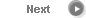#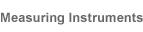# Precise Color Communication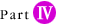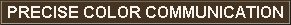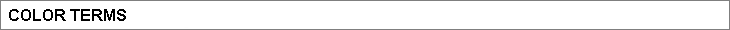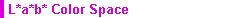The L*a*b* color space (also referred to as the CIELAB space) is one of the uniform color spaces defined by the CIE in 1976. The values of L*, a*, and b* are calculated according to the formulas below: Lightness variable L*: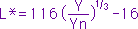Chromaticity coordinate a* and b*: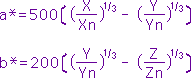where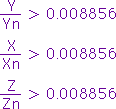X, Y, Z: Tristimulus values XYZ (for 2 Standard Observer) or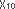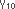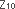(for 10 Standard Observer) of the specimen Xn, Yn, Zn: Tristimulus values XYZ (for 2 Standard Observer) or(for 10 Standard Observer) of a perfect reflecting diffuser If X/Xn, Y/Yn, or Z/Zn is less than 0.008856, the above equations are changed as described below: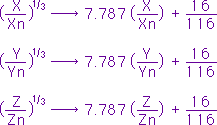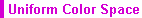A color space in which equal distances on the coordinate diagram correspond to equal perceived color differences.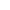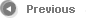4/12